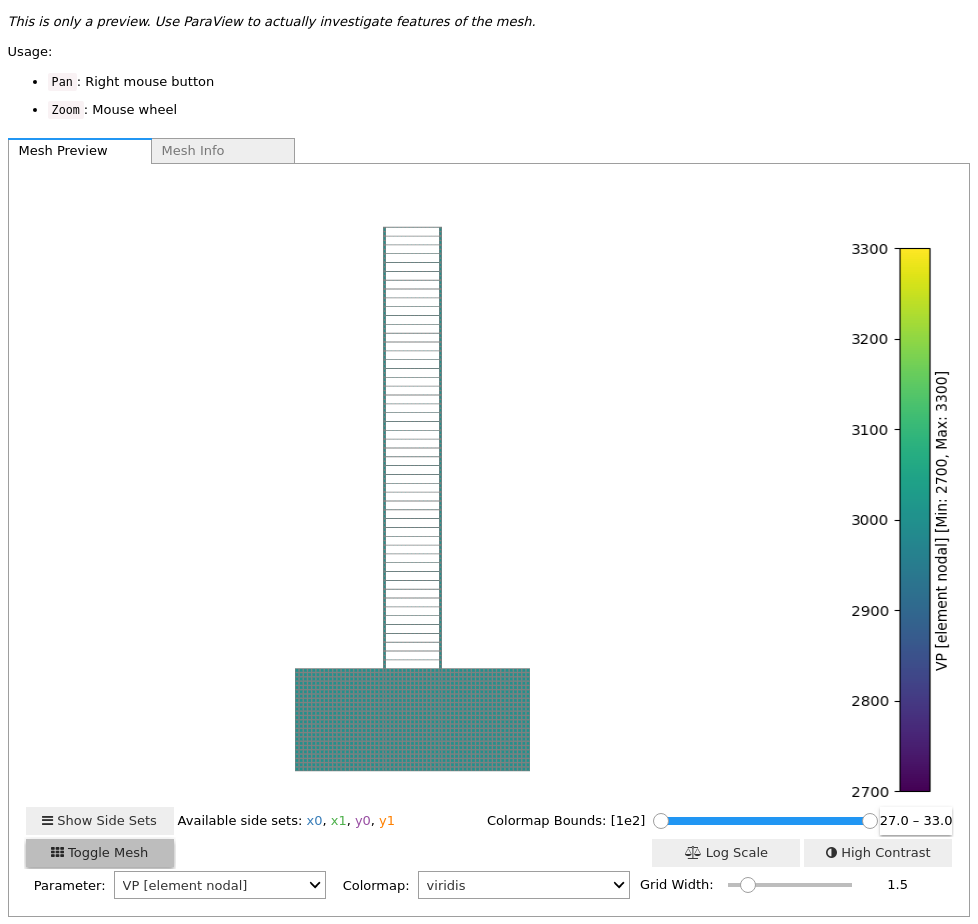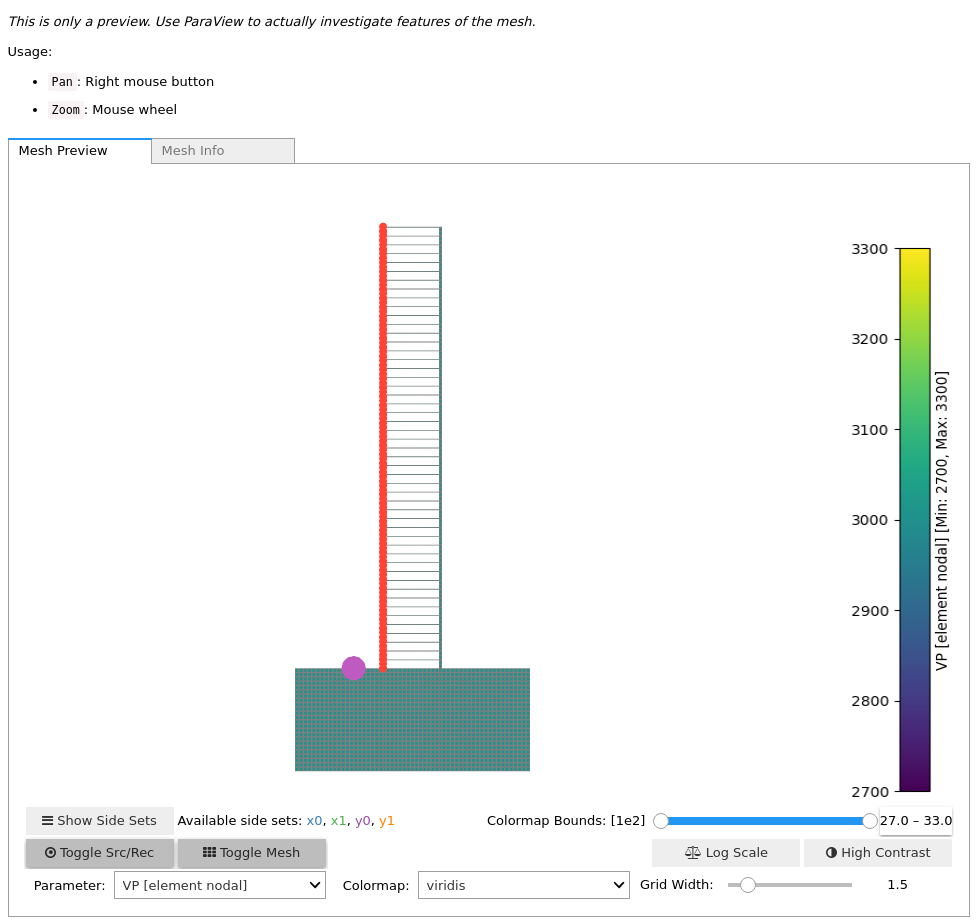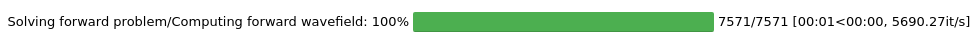Version:

This documentation is not for the latest stable Salvus version.This tutorial is presented as Python code running inside a Jupyter Notebook, the recommended way to use Salvus. To run it yourself you can copy/type each individual cell or directly download the full notebook, including all required files.Ground Motion Modelling in Buildings

Copy
# Magic
%matplotlib inline
%config Completer.use_jedi = False

Introduction

In this tutorial we will use Salvus Mesh to generate a simplified model of a "building", and instrument the resulting model with a series of sensors which record the gradient of displacement at a series of discrete points. Specifically, we will:
• Use the SalvusToolbox to generate a parameterized model of a building.
• Attach a homogeneous set of material parameters to the resulting mesh.
• Instrument the left wall with a series of sensors which will record the gradient of displacement.
• Run a simluation.
• Plot and analyze the results.
import os
import matplotlib.pyplot as plt
import numpy as np

import salvus.flow.simple_config as config
import salvus.toolbox.toolbox as st
import salvus.toolbox.toolbox_geotech as st_geo
from salvus.flow import api

SALVUS_FLOW_SITE_NAME = os.environ.get("SITE_NAME", "local")
--> Server: 'https://data.mondaic.com/license_server/licensing_server', User: '_MONDAIC_INTERNAL_', Group: '_MONDAIC_INTERNAL_'.
--> Negotiating 1 license instance(s) for 'SalvusMesh' [license version 1.0.0] for 1 seconds ...
--> Success! [Total duration: 1.01 seconds]

Generating the mesh

To generate our idealized building we'll use a function internal to the Salvus toolbox -- if you're curious how it works, you could take a look at the get_simple_building function we've imported above. The function takes a variety of values which parameterize how the mesh is generated. The values below produce a 50 "story" building with a basement foundation. As with the rest of Salvus, all units are SI.
n_stories = 50
wall_width = 1.0
story_height = 3.0
ceiling_height = 0.3
building_width = 20.0
basement_depth = 20.0
basement_width = 50.0
Before we generate the mesh itself we need to set a few values which determine the resolution of the resulting simulation. For a more detailed discussion on the proper values to choose here, please see one of the other tutorials, such as the "Marmousi" tutorial. Below we'll assume a constant shear-wave velocity value of $1500$ m/s, and, using this as the minimum velocity in the mesh, mesh the domain so that we have at least 2 elements per wavelength assuming a maximum frequency of $500$ Hz.
f_max = 500.0
vs_min = 1500.0
nelem_per_wavelength = 2.0
Now its time to build the mesh itsef. Below we pass the requisite parameters to the generation function, and receive back a the tuple: (mesh, bnd). The first entry here is indeed our mesh -- we'll plot it in a few cells -- and the bnd value represents the minimum distance to which the mesh was extruded to attach absorbing boundary layers. This value will be helpful later when we're setting up the simulation.
mesh, bnd = st_geo.get_simple_building(
f_max=f_max,
vs_min=vs_min,
n_stories=n_stories,
wall_width=wall_width,
story_height=story_height,
basement_width=basement_width,
basement_depth=basement_depth,
building_width=building_width,
ceiling_height=ceiling_height,
nelem_per_wavelength=nelem_per_wavelength,
)
Now that we've got the mesh skeleton, we can simply attach our elastic parameters as shown below. We'll use the vs_min value we defined above for our shear wave velocity, and use a simple scaling relation such that the p-wave velocity is twice the s-wave velocity. These parameters of course can be changed, and can also be made heterogeneous, but one must always keep in mind the resolution criterion (here: 2 elements per wavelength given a minimum velocity of $1500$ m/s) in mind.
nodes = mesh.get_element_nodes()[:, :, 0]
mesh.attach_field("RHO", np.ones_like(nodes) * 1000)
mesh.attach_field("VS", np.ones_like(nodes) * vs_min)
mesh.attach_field("VP", np.ones_like(nodes) * vs_min * 2)
With our parameters attached, we can now plot the model below.
mesh<salvus.mesh.unstructured_mesh.UnstructuredMesh at 0x7f1a55f52890>

Setting up the simulation

Here we set up a simple source to inject some energy for the upcoming simulation. We use here a Ricker source-time function with a center frequency which is half the maximum frequency in the mesh. More information on the available source-time functions and source types can be found in the relevant tutorials.
stf = config.stf.Ricker(center_frequency=f_max / 2)
source = config.source.cartesian.VectorPoint2D(
x=-20, y=0.0, fx=1.0, fy=1.0, source_time_function=stf
)
Now we set up an array of 100 sensors which will travel up the left wall of our building. In addition to setting the spatial locations for each receiver, we'll also tell Salvus to output the velocity and gradient of displacement at each spatial location.
n_rec = 100
x_rec = np.ones(n_rec) * -building_width / 2
y_rec = np.linspace(0, n_stories * story_height, n_rec)
x=x,
y=y,
station_code=f"{_i:03d}",
)
for _i, (x, y) in enumerate(zip(x_rec, y_rec))
]
Now we can set up our simulation object, which acts as the parameter file to send to Salvus. We attach the absorbing boundaries at the relevant side-sets, give our simulation a physical end time, and also specify what type of output we want. The volumetric output is commented out below, as this can take up a sizeable chunk of disk space depending on your simulation parameters. Feel free to uncomment this if you have plenty of space -- it is directly from this output that the movie at the end of this tutorial was generated.
# Initialize with sources and receivers.

# Set end time, start time is automatically determined.
w.physics.wave_equation.end_time_in_seconds = 0.1

# Define and attach our absorbing boundaries.
ab = config.boundary.Absorbing(
side_sets=["x0", "x1", "y0"], width_in_meters=bnd, taper_amplitude=f_max
)
w.physics.wave_equation.boundaries = [ab]

# Use simplified HDF5 source output.
w.output.point_data.format = "hdf5"

# Save the volumetric wavefield for visualization purposes.
# w.output.volume_data.format = "hdf5"
# w.output.volume_data.filename = "output.h5"
# w.output.volume_data.fields = ["displacement"]
# w.output.volume_data.sampling_interval_in_time_steps = 10

# Ensure that Salvus will accept our parameters.
w.validate()

# Plot the mesh with the sources and receivers.
w<salvus.flow.simple_config.simulation.Waveform object at 0x7f1a44e89410>
With everything defined, plotting the simulation.Waveform() object gives us a visual representation of where our sources and sensors were placed. As expected, we've instrumented the left wall with a series of velocity / gradient sensors, and placed a source just outside the building.

Running the simulation

Running the simulation is as simple as calling the api.run command as below. This simulation is rather small, so running on 2 ranks locally should allow for the simulation to complete within 10 - 30 seconds. Of course for bigger simulations (or simulations in 3D) we could install Salvus on a remote supercomputing site. In this case, none of the above workflow would change, and all we would do is specify the remote site for our site_name below. Salvus Flow would take care of shuttling data to and from the remote machine as required.
api.run(
ranks=2,
get_all=True,
input_file=w,
overwrite=False,
site_name=SALVUS_FLOW_SITE_NAME,
output_folder="output",
)
Job job_2110191023273492_697a77f7b3 running on local_cuda with 2 rank(s).
Site information:
* Salvus version: 0.11.42
* Floating point size: 32* Downloaded 17.4 MB of results to output.
* Total run time: 3.18 seconds.
* Pure simulation time: 2.67 seconds.
<salvus.flow.sites.salvus_job.SalvusJob at 0x7f1a433fccd0>

Analyzing the results

Below we plot a "shotgather" showing the output of the displacement gradient sensors we placed along the left wall of the building. While informative in their own right, such results could be used to also investigate the rotational motion recorded at each sensor if desired.
f, ax = plt.subplots(2, 2, figsize=(15, 10), sharex=True, sharey=True)